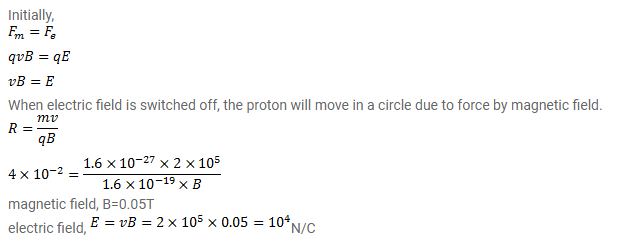# A proton goes undeflected in a crossed electric and magnetic field

Question:

A proton goes undeflected in a crossed electric and magnetic field (the fields are perpendicular to each other) at a speed of $2.0 \times 10^{5} \mathrm{~m} / \mathrm{s}$. The velocity is perpendicular to both the fields. When the electric field is switched off, the proton moves along a circle of radius $4.0 \mathrm{~cm}$. Find the magnitudes of the electric and the magnetic fields. Take the mass of proton $=1.6 \times 10^{-27}$ $\mathrm{kg}$.

Solution: